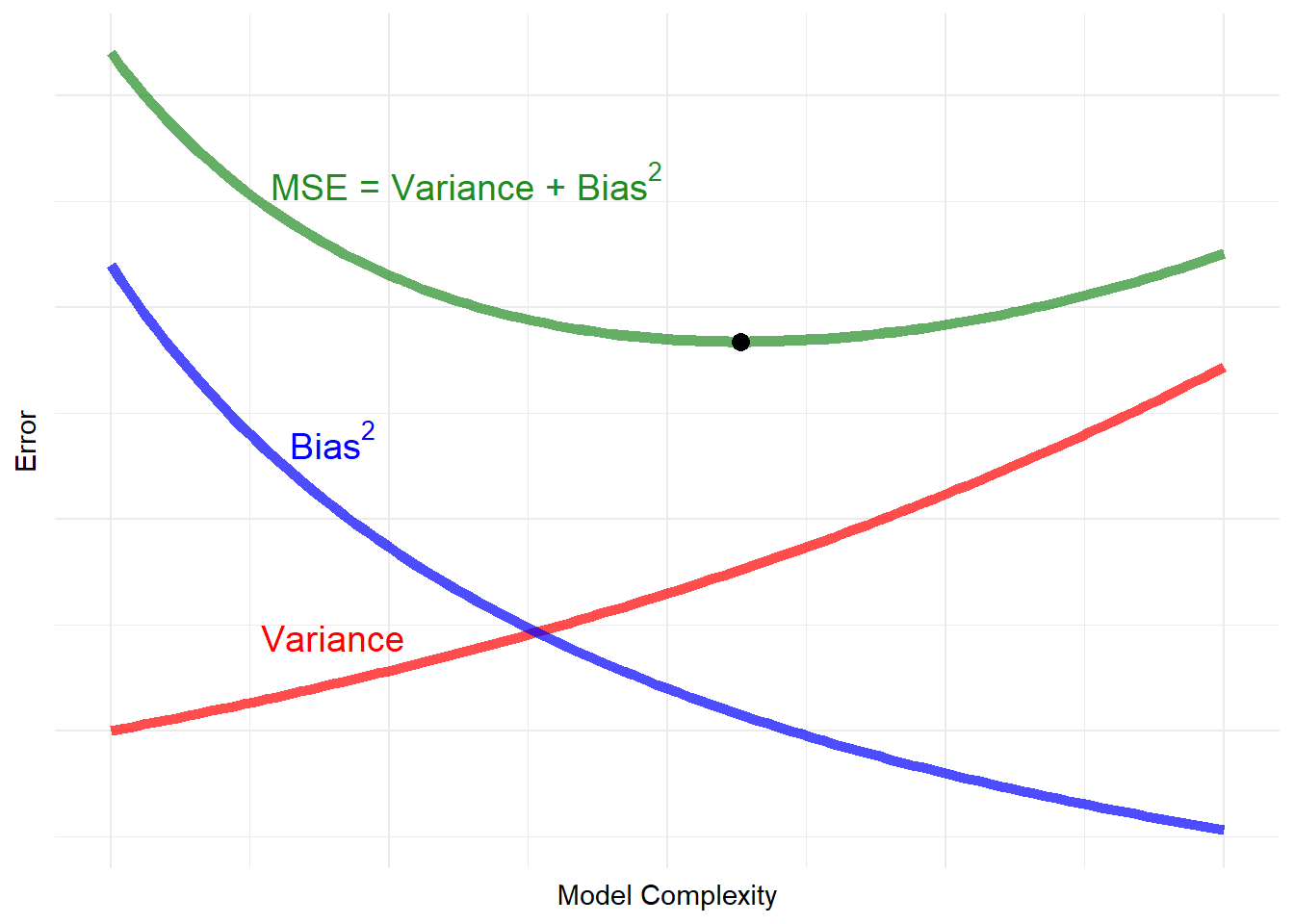# Articles by R on Doruk Cengiz's site

### Double Descent Part I: Sample-wise Non-monotonicity

July 12, 2021 |

One of the most fascinating findings in the statistical/machine learning literature of the last 10 years is the phenomenon called double descent. For some reason, extremely over-parameterized models, where the number of parameters to estimate is 10-...### The Simple Logic Behind the $\widehat\beta$ of the OLS
The (in)famous OLS estimator, $$\widehat\beta = (X^{T}X)^{-1}X^{T}y$$. When people see this formula for the first time, the first reaction is to assume that it came from complex mathematical proofs, so it is OK if it’s not very intuitive. While it i... [Read more...]